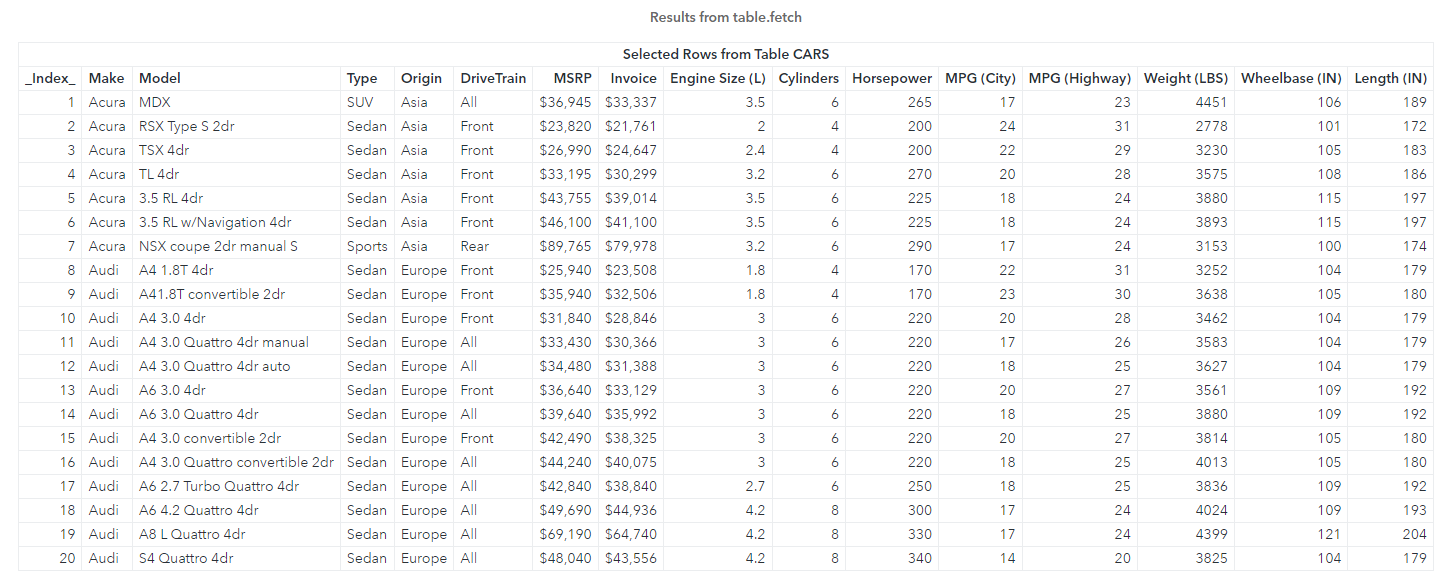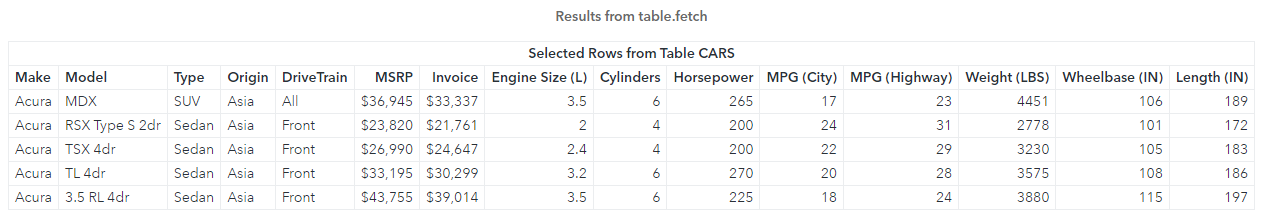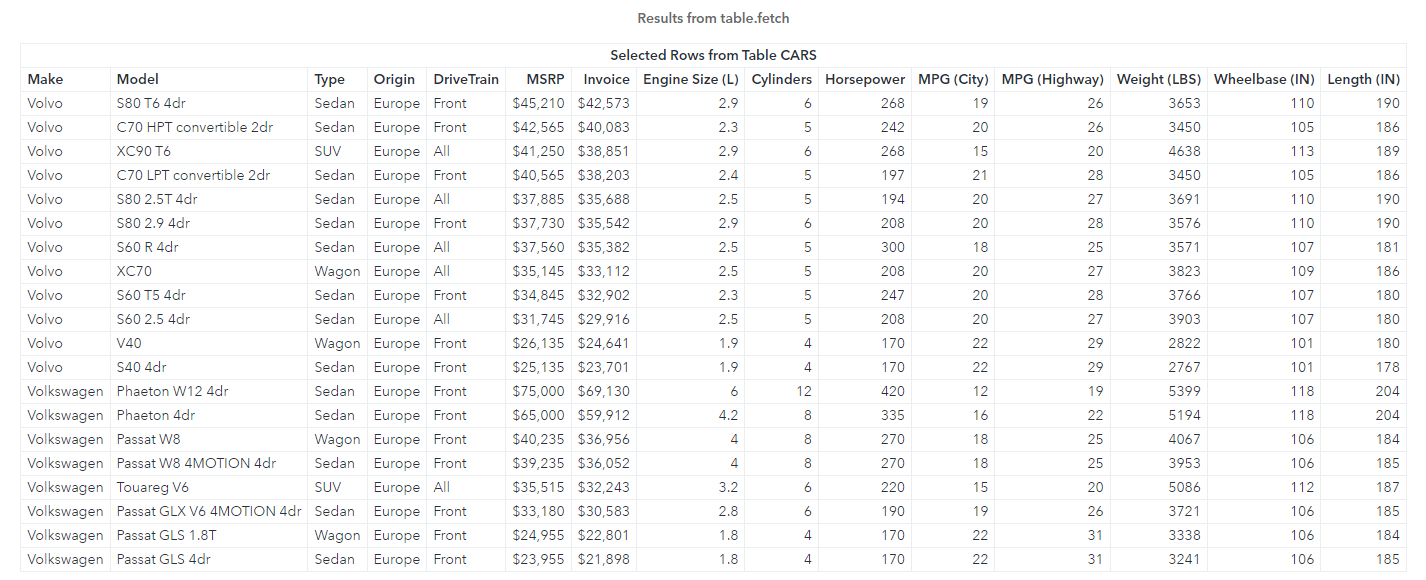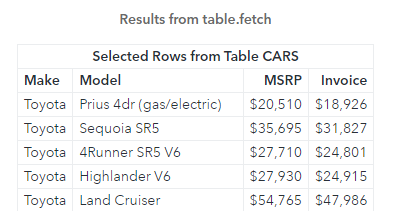Welcome to my SAS Users blog series CAS Action! - a series on fundamentals. I've broken the series into logical, consumable parts. If you'd like to start by learning a little more about what CAS Actions are, please see CAS Actions and Action Sets - a brief intro. Or if you'd like to see other topics in the series, see the overview page. Otherwise, let's dig in with our first topic - the fetch action.

The table.fetch CAS action retrieves the first 20 rows of a CAS distributed in-memory table. It's similar to using the head method in R or Python. However, the fetch action can do more than fetch rows from a table. In this example, I will use the CAS procedure to execute the fetch action. Be aware, instead of using the CAS procedure, I could execute the same action with Python, R and other languages with some slight changes to the syntax. Pretty cool!

## Retrieve the first 20 rows of a CAS table using the fetch action

To begin, let's use the table.fetch CAS action on the cars in-memory table to view the action's default behavior. Consider the following code:

```proc cas; table.fetch / table={name="cars", caslib="casuser"}; quit;```

To use the fetch action with the CAS language, you specify the CAS procedure, the action, a forward slash, then the table parameter to specify the CAS table. In the table parameter I'll use the name sub-parameter to specify the cars in-memory table, and the caslib sub-parameter to specify the casuser caslib. The result of the call is listed below.We retrieved 20 rows from our distributed cars table. What else can we fetch?

## Retrieve the first n rows of a CAS table

What if you don't want the first 20 rows? Maybe you only want the first 5. To modify the amount of rows returned use the to parameter, then specify the number of rows. In the results, I also saw an _Index_ column. That column appears by default. To remove the _Index_ column, add the index=FALSE parameter.

```proc cas; table.fetch / table={name="cars", caslib="casuser"}, to=5, index=FALSE; quit;```The results return 5 rows from the cars table, and the _Index_ column has been removed.

Now, what if I want to sort the returned rows?

## Sort the table

The results of a CAS table are not guaranteed since the table is distributed among the CAS workers. To see the results in a precise order, use the sortBy parameter. The sortBy parameter requires an array of key-value pairs (dictionaries), so it's a bit tricky the first time you use it.

In this example, let's sort the table by Make and MSRP in descending order.

```proc cas; table.fetch / table={name="cars", caslib="casuser"}, sortBy={ {name="Make", order="DESCENDING"}, {name="MSRP", order="DESCENDING"} }, index=FALSE; quit;```The results show 20 rows of the cars table sorted by Make and MSRP. Great!

## Subset the table

What if I only want to see the cars where Make is Toyota, and return the columns Make, Model, MSRP and Invoice? You can add the where and vars sub-parameters in the table parameter to subset the table.

```proc cas; table.fetch / table={name="cars", caslib="casuser", where="Make='Toyota'", vars={"Make","Model","MSRP","Invoice"} }, to=5, index=FALSE; quit;```The results return 5 rows from the cars table where Make is Toyota and only the four specified columns.

### Quick detour

Instead of using the vars sub-parameter as we did in the previous example, you can use the fetchVars parameter. The code would change to:

```proc cas; table.fetch / table={name="cars", caslib="casuser", where="Make='Toyota'"}, fetchVars={"Make","Model","MSRP","Invoice"}, to=5, index=FALSE; quit;```

Either method works and it's totally up to you.

## Create a calculated column

Lastly, let's create a calculated column named MPG_Avg that calculates the average city and highway miles per gallon for each car, then subset the results for all cars with an MPG_Avg greater than 40. To create a calculated column, use the computedVarsProgram sub-parameter in the table parameter. Then you can subset on the calculated column with the where sub-parameter.

```proc cas; table.fetch / table={name="cars", caslib="casuser", vars={"Make","Model","MPG_Avg"}, where="MPG_Avg > 40", computedVarsProgram="MPG_Avg=mean(MPG_City,MPG_Highway)" }, sortBy={ {name="MPG_Avg", order="DESCENDING"} }, index=FALSE; quit;```

## Summary

CAS actions are optimized to run on the CAS server on large data. They are flexible and offer many parameters to control the output and can be executed in a variety of languages! In the next post, I'll cover more on the fetch action.